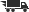Close

# Introduction to Mathematical Programming

## by Russell C. Walker

• ISBN: 9780132637657
• ISBN10: 0132637650

# Introduction to Mathematical Programming

## by Russell C. Walker

• List Price: \$73.33
• Binding: Hardcover
• Edition: 1
• Publisher: Prentice Hall
• Publish date: 12/01/1998
• ISBN: 9780132637657
• ISBN10: 0132637650
used \$12.98
You save: 82%
Description: "Empowering users with the knowledge necessary to begin using mathematical programming as a tool for managerial applications and beyond, this practical guide shows when a mathematical model can be useful in solving a problem, and instills an appreciation and understanding of the mathematics associated with the applied techniques. "Surveys problem types, and discusses various ways to use specific mathematical tools. Contains prerequisite material for the study of linear programming, and offers a brief introduction to matrix algebra. Discusses the special structures of four network problems: the transportation problem, the critical path method, the shortest path problem, and minimal spanning trees. Covers compound interest and explores the financial aspects of specific problems considered throughout the book. Touches on "mathematics" oriented (vs. applications) material, with integrated proofs and discussions on such topics basic graph theory, linear algebra, analysis, properties of algorithms, and combinatorics. An extensive appendix section includes answers to many problems, an introduction to the linear programming package LINDO, an overview of the symbolic computation package "Maple," and brief introductions to the "TI-82" and "TI-92" calculators and their applications.
Expand description
 Seller: One Stop Shop Bookstore ★★★★★ ★★★★★ Location: Memphis, TN Condition: GoodPrice: \$41.00 Comments: